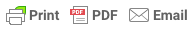Sorry, you do not have permission to ask a question, You must login to ask question. Become VIP Member

Deduction is necessary in certain cases while estimations are carried out for masonry constructions. For instance, the presence of openings in masonry walls, bearings, doors, windows, segmental arch openings, and semi-circular arch openings. Deductions for these conditions shall be conducted properly and the suitable mathematical rule needs to be applied.

## 1. Rectangular Opening

Commonly, full reduction is done for the rectangular openings during estimation of masonry construction. The deduction quantity is equal to the opening height times its width times the thickness of the masonry wall.

## 2. Doors and Windows with Small Segmental Arch

In this case, deduction is made for the rectangular portion of the door or window up to the springing line. The computation of deduction amount is similar to that of the rectangular opening discussed above. The segmental arch is assumed to be solid to allow for possible extra cost used in the construction of the arch.

## 2. Segmental Arch Openings

Deduction is done for the entire opening which includes rectangular and segmental part. The reduction quantity is estimated as follow:

Deduction quantity= b*h+2/3*b*r*wall thickness          Equation 1

## 3. Semi-Circular Arch Opening

The actual area for semi-circular portion is computed as follow:

Area=0.5*PI*r^2              Equation 2

However, the amount of reduction which is made for semi-circular part is computed using the following expression:& Ad Free!

GET VIP MEMBERSHIP NOW!

Area=3/4*b*r                 Equation 3

And the total deduction quantity for the entire opening is calculated using the following formula:

Area=b*h+ 3/4*b*r        Equation 4

As for elliptical arches, it is considered as semi-circular arches and the deduction quantity estimation is the same.

## 4. Lintels Over Openings

Lintels Over Openings is another Item that needs to be deduced from the masonry construction estimation. The deduced quantity which the volume of the lintel is calculates using the following equation:

Deduced Quantity= Lintel Length* Thickness* Wall Thickness  Equation 5

The length of the lintel is equal to the lintel span plus two times lintel bearing. If the dimension of the bearing is not provided, then a minimum bearing length of 12 cm can be assumed.

## 5. Arch masonry work

It is another element that should be removed from the masonry construction estimation. The volume of the arch as shown in Fig. 5 is calculated by multiplying the mean length of the arch by its thickness by the width of the wall.

EDITOR
Madeh is a Structural Engineer who works as Assistant Lecturer in Koya University. He is the author, editor and partner at theconstructor.org.

## How to Reduce the Possibility of Crack Development in Concrete?

1.